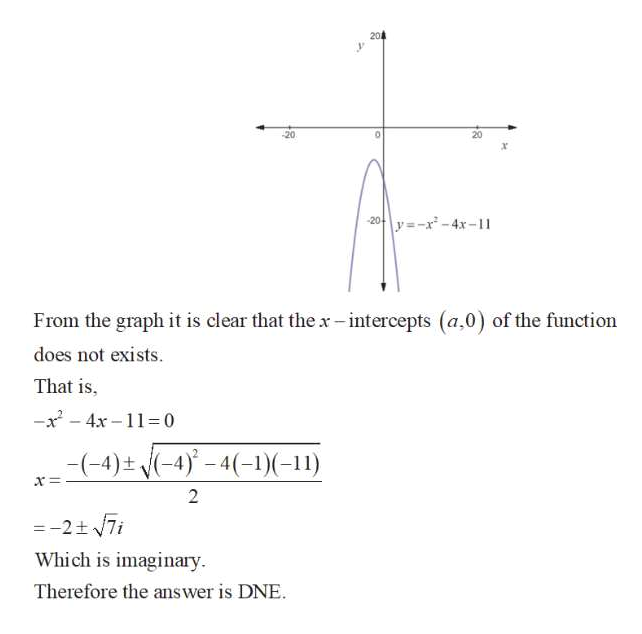# Find the coordinates of the x-intercepts of the graph of the equation. (If an answer does not exist, enter DNE.)y = −x2 − 4x − 11(x, y) =      Find the minimum or maximum value of the quadratic function.f(x) = −4x2 + 8x − 6 ---Select--- minimum value maximum value     f(x) =

Question
1 views

Find the coordinates of the x-intercepts of the graph of the equation. (If an answer does not exist, enter DNE.)

y = −x2 − 4x − 11
(x, y) =

Find the minimum or maximum value of the quadratic function.

f(x) = −4x2 + 8x − 6

---Select--- minimum value maximum value

f(x) =
check_circle

Step 1

The given equation is,

Step 2help_outlineImage Transcriptionclose204 -20 20 -20-y=-x -4x-11 From the graph it is clear that the x- intercepts (a,0) of the function does not exists. That is, -x - 4x –11= 0 -(-4) ± j(-4)° – 4(-1)(-11) =-2+ 7i Which is imaginary. Therefore the answer is DNE. fullscreen

### Want to see the full answer?

See Solution

#### Want to see this answer and more?

Solutions are written by subject experts who are available 24/7. Questions are typically answered within 1 hour.*

See Solution
*Response times may vary by subject and question.
Tagged in

### Other